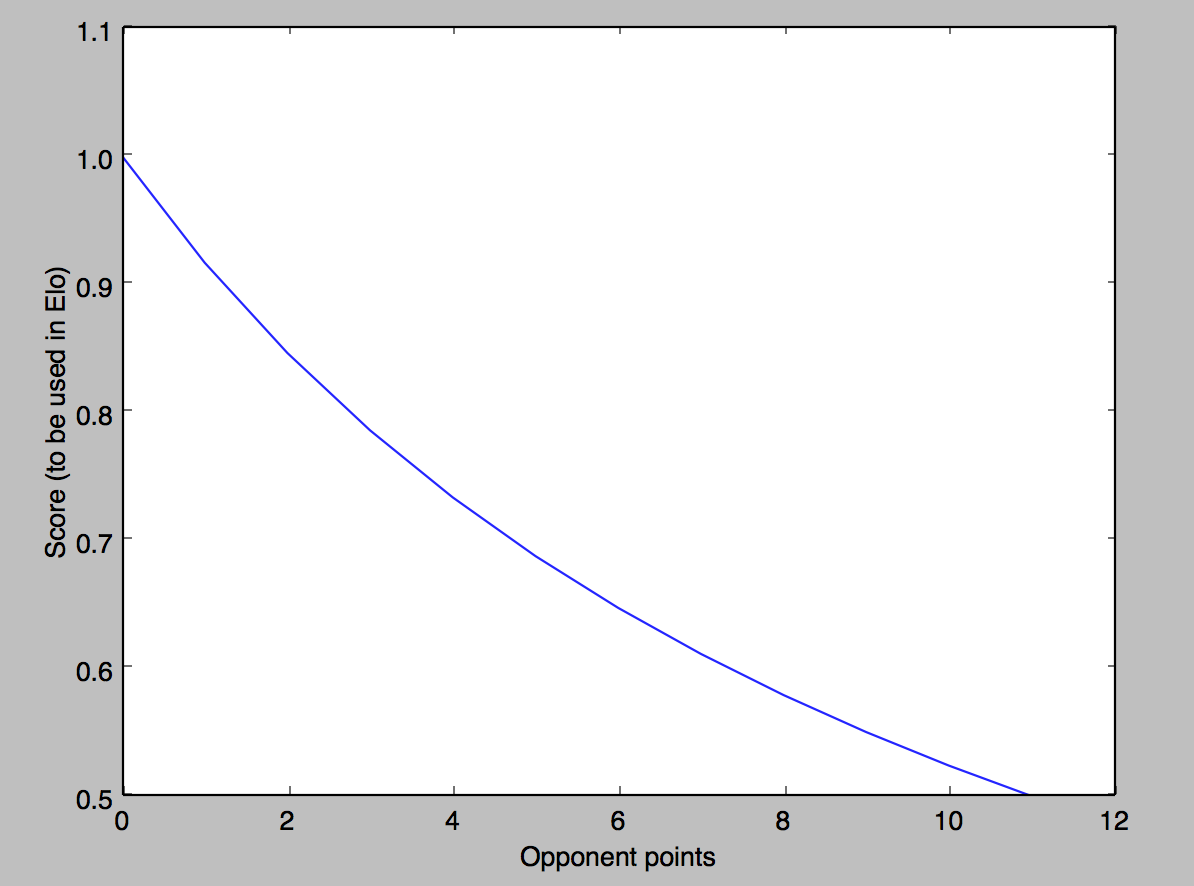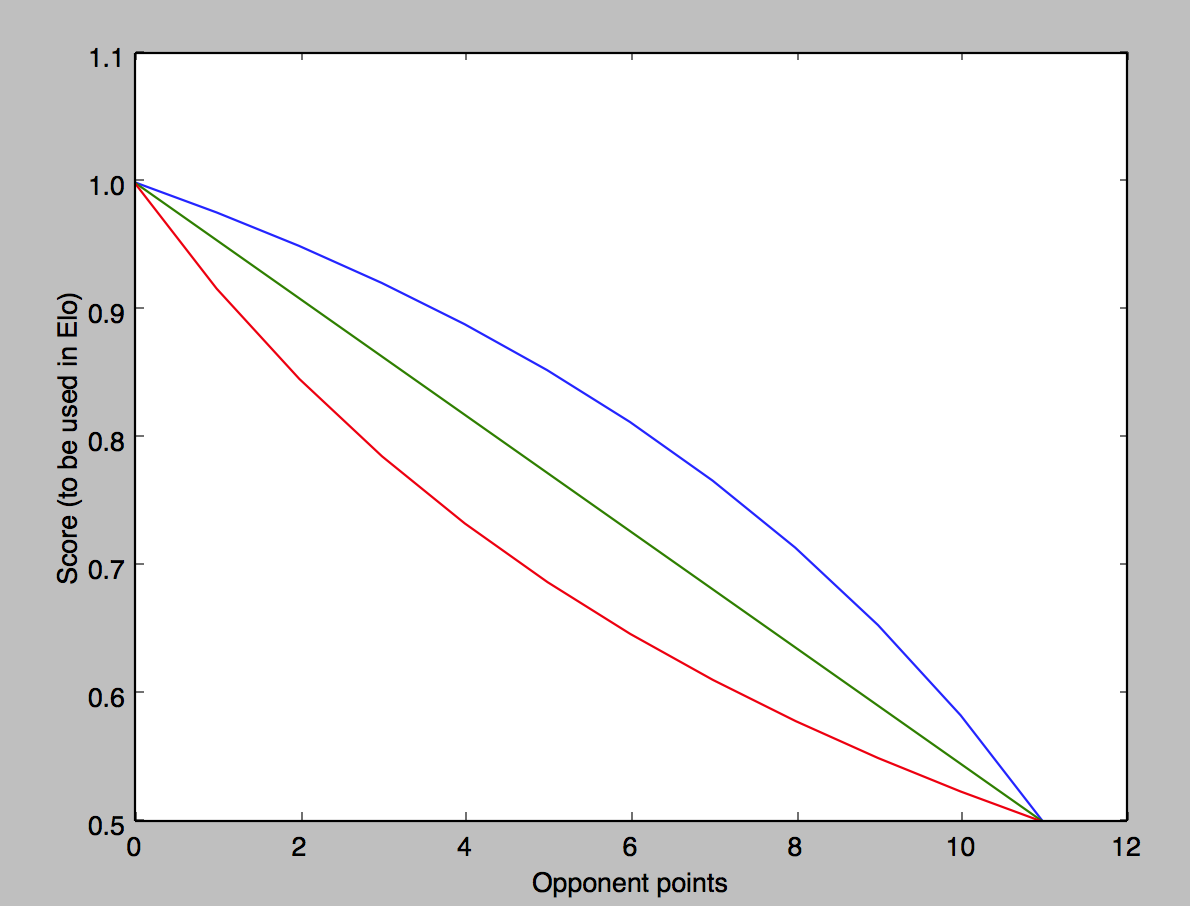A rating system for table tennis

## Background

At work, during breaks, most of my team plays table-tennis. This weekend, I decided to spend some time writing up a rating system that I could use to keep track of how good (or bad) I (and everyone else) was. This is a writeup of how that exercise went. I’ve hidden a few lines to act as spoiler (indicated by an arrowhead to the left of the line). If you wish to exercise your mathematical skills, try making a guess and then click the line to reveal how I solved the problem.

## The aim

A good rating function should ideally be able to be accurately predict who would win a game between two opponents. Since an ideal rating function seems impossible to achieve, I decided to build a rating system based on Elo (a popular rating system used in chess and unofficially in some other sports).

## Elo

Elo has spawned many rating systems, including Glicko and other variations used in e-sports. Elo as used in chess gives a score of one to the winner and zero to the loser of each game. In case of a tie, both players get a score of 0.5 each.

## Setting goals

This clearly requires some modifications. Specifically, we will modify how the score is awarded. Firstly, there are no ties in table tennis. There is always a winner and a loser, so that gives us a simple property we can require our rating function to have.

Property 1: The winner of each game should gain rating points while the loser should lose rating points.

Initially, I thought that given we are basing the system on Elo, the winner of each game should get a score > 0.5 and a loser should score < 0.5. Since these are disjoint, it also ensures that the winner’s score is always greater than the loser’s score.

However, this turns out to not to satisfy Property 1 because: you can score lower than your expected score (as predicted by Elo), even if you score more than 0.5. This would cause you to lose rating points.

We can also easily see another desirable property the score should have.

Property 2: A win with a larger po difference should lead to a larger rating increase than one with a smaller score difference (other factors remaining constant).

The “other factors remaining constant” here refers to the fact that a rating increase/decrease when working with Elo will depend on both opponents’ initial ratings. The second property would require a 11-3 win to garner the victor a bigger rating increase than a 11-7 win. Notice that this places no restrictions on how the rating increase should decrease as the loser scores more points. Specifically, we don’t tie ourselves to saying that a 11-3 win versus a 11-2 win should see the same decrease in rating increase that a 11-4 win versus a 11-3 win would see. In other words, the function need not be linear.

This lets us arrive at our first way to calculate the score: ``` player_1_score = player_1_points/(player_1_points + player_2_points) ```

This function satisfies both of the above properties. It also highlights another interesting aspect of games like table tennis - the total number of points is unbounded (due to tiebreaks). Theoretically, you could have a game that ends 999999-999997, although this is highly unlikely (proof left as exercise to reader). However, games can end 17-15, 15-13, etc. often enough to be considered. This means we cannot assume a fixed “maximum total number of points” to use in our rating function (the existence of such a metric would have allowed us to get nice, linear functions). Also, this gives us another property we would require. A “closer” game (17-15) should give fewer points to the winner than one that is not as close (12-10), even if the point difference is the same. Concretely:

Property 3: In games with the same score difference, the one with the higher point total should lead to a smaller rating increase.

Now, let us graph the score function we mentioned above.We can see now that the function has at least one annoying feature. If you win a game 11-0, then you get a score of 1. But if you win a game 11-3, you get a significantly smaller score. While a question of taste, I would consider both of these to be decisive victories, worthy of scores close to 1. The function I want to model should ideally hover around 1 for low values of opponent’s score (x axis) and then drop towards 0.5 when an opponent has lost the game only by a few (2-3) points. Two functions come to mind that would have this property:

1) The sigmoid function (if you have any ML experience you should know what the graph of this looks like)
2) The reflection of the function we already have across the line that connects its endpoints.

## Reflecting across a line

I started off with the sigmoid and then spent two hours trying to figure out how to reflect a line. My mathematics is a bit rusty, so if you have a better (or an alternative) method, please do share! First, I had to find the equation of the line connecting the end-points. That’s pretty simple - the y-intercept is 1 and the x-intercept is 11 (or 21) times 2 since the line extends 11 points for half the y-axis (0.5 to 1).

That means the equation of the line is: ``` x/22 + y/1 = 1 y = 1 - x/22 line(x) = 1 - x/22 ```Now, to reflect the function across this line. I really struggled with this - not sure if we learned it in school, but I didn’t want to look it up. I finally did it by considering a point on the x-axis (let’s say point A) where we want to plot a point of the reflected function somewhere on the y dimension. We can “drop a perpendicular” at point A. This line will intersect both the reflected point as well as the line y = 1 - x/22. If we drop another perpendicular at the intersection of the line and the first perpendicular, then we should intersect the curve representing our function at the point to be reflected.

In other words, we need to find the value of x using: ```f(x) = line(A) [where x is the opponent's score] player_points/(x + player_points) = line(A) player_points/line(A) = x + player_points player_points/line(A) - player\_points = x player_points*(1/line(A) - 1) = x x = player_points*(1/line(A) - 1) x = player_points*(22/(22-A) - 1) x = player_points*(22 - 22 + A)/(22-A) x = player_points*A/(22-A) ```

Once we have the value of x,

the location of the point is given by the x-y coordinates: A, line(x)

Now, to get the reflected line, we plot line(x) for every value of opponent_score (the x axis), getting the following graph:I now know this can be expressed as a much simpler function (although I just found it coincidentally - I didn’t come to it by attempting to find a reflection).

This can be done by using the opponent's score: 0.5 + opponent_score/(opponent_score + player_score) </summary>

On the bright side, I guess I got to think about reflecting across a line. Oh well.

## The sigmoid

For the sigmoid, I used the reflected function as the input. The reflected function needed to be offset and scaled before it could be used as the input. Au gout du jour, I did this by randomly tweaking parameters till the graph looked fairly alright. I wanted the dip to be when the opponent had won around 70% of points (7-8 points out of 11). Then, I wanted it to drop steeply to near 0.5 around 9.

Since the sigmoid gives a result between 0 and 1, I had to divide by 2 and add 0.5 to push it up the y-axis. Done!Well, not really. This turns out to fail Property 1 (as stated right after Property 1) because while the score may be > 0.5 for a winner, the expected score may be even higher. To solve this, I scaled the output of the sigmoid to be between 1 and the expected Elo and then offset it.

If O is the output of the sigmoid, this can be done by the equation: O*(1-expected_Elo) + expected_Elo

Since 0.5 effectively represents a tie, I used max(0.5, expected_Elo) instead. This seems to work fairly well.

## Other issues (or “Future work”)

With the current function, we probably want the dropoff to move with the expected_Elo (as the expected_Elo goes to 1, we want the dropoff to move to the left). Since we don’t want it to move further than let’s say around 4 (in a game to 11), only games that have scores between 4-8 will be affected. I haven’t calculated how much the scores will be off by.

Another property we would like to have (but I haven’t checked for) is:

Property 4: Games to 21 points should be weighted more heavily in the ratings compared to games to 11 points.

## Other approaches

I looked at Glicko, but looks like the only improvement that introduces over Elo is that it introduces a rating deviation parameter. The parameter affects how much a rating changes (it would cause it to change less when a player has played only a few games as their rating is not well established then).

I also tried using points as the equivalent of matches in chess. However, that ended up not satisfying Property 1.

## Reflections (pun unintentional)

At the end of it all, I learned a lot. Scaling functions, visualizing various mathematical approaches & methods while trying to get to grips with an unfamiliar function that is now a tad more familiar (Elo) made this fun. Nothing like coming to conclusions on your own :)